## Volume

Let’s revisit our friend Wally from “Area and Perimeter” and use another aspect of his yard to introduce the concept of volume. Wally is a swimmer and wants to install a lap pool in his backyard. Because he has some extra space, he is going to build a pool that is 25 yards long, 2 yards wide, and 2 yards deep. How many cubic yards of water must be used to fill the pool (assuming right to the top).

Much as we did with area (counting unit squares), with volume we will be counting unit cubes. What is the volume of a unit cube? Let’s look at figure 1:

### Finding the Volume of a Cube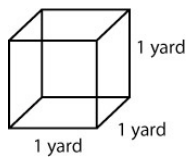Figure 1. Volume = 1 yd × 1yd × yd = 1 cubic yard

The shape at left is a cube (all sides are equal length). In particular, because all sides are of length 1, this cube is called a unit cube.

We know the area of the base from our previous work (1 yd × 1 yd or 1 square yard). We are going to take that area and extend it vertically through a height of 1 yard so our volume becomes

Volume = 1 yd × 1yd × yd = 1 cubic yard

How does this help Wally? Well, if he can count the number of unit cubes in his pool, he can determine the volume of water needed to fill the pool.

### Guided Example

#### Filling Wally’s Pool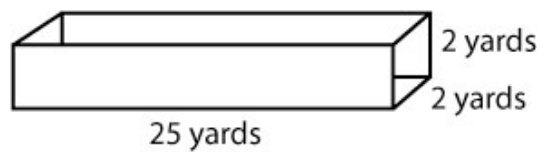Figure 2. Volume = Length × Width × Height or V = LWH

If we fill the pool with unit cubes, we can fill 25 unit cubes along the length, 2 along the width and 2 along the height. That would give us 25 × 2 × 2 = 100 unit cubes or:

Volume = 25 yd × 2 yd × 2 yd = 100 cubic yd

Explicit formulas for the types of rectangular solids used in the previous section are as follows:

Shape Volume
Cub of side length L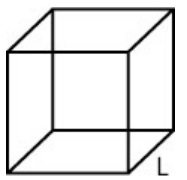$V=L\times{L}\times{L}\\$
$V=L^3\\$
Box with sides of length L, W, H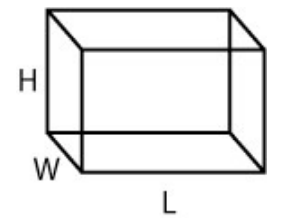$V=L\times{W}\times{H}\\$

### Notes on Volume

• Volume is a three-dimensional measurement that represents the amount of space inside a closed three-dimensional shape.
• To find volume, count the number of unit cubes inside a given shape.
• If there are units, include units in your final result. Units will always be three-dimensional (i.e. cubic feet, cubic yards, cubic miles, etc…)

### Example 1

Find the volume of each shape below.

1.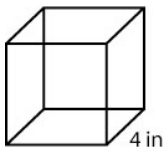2. A box with sides of length 2 ft, 3 ft, $\displaystyle{2}\frac{1}{2}\\$ ft.

1. 64 in3
2. 15 ft3

## Volume of A Circular Cylinder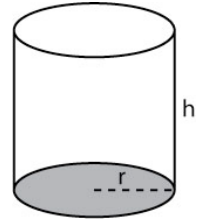Figure 3.

Can we use what we know about the area of a circle to formulate the volume of a can (also called a cylinder)? Take a look at figure 3.

The base circle is shaded. If we take the area of that circle (A = A = πr2) and extend it up through the height h, then our volume for the can would be:

V = πr2h.

### Example 2

Find the volume of the cylinder shown below.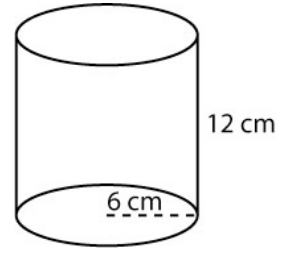432π cm3

## Volume of Other Shapes

The chart below shows the volumes of some other basic geometric shapes.

Shape Volume
Sphere $\displaystyle{V}=\frac{4}{3}\pi{r}^3\\$
Cone $\displaystyle{V}=\frac{1}{3}\pi{r}^2h\\$
Pyramid $\displaystyle{V}=\frac{1}{3}LWH\\$

### Example 3

Find the volume of a sphere with radius 5 meters.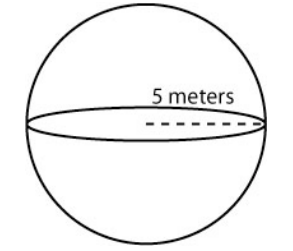#### Solution

$\displaystyle\frac{500}{3}\pi\text{ cm}^3\\$

### Example 4

Determine the volume of each of the following. Include a drawing of the shape with the included information. Show all work. As in the examples, if units are included then units should be present in your final result. Use 3.14 for π and round answers to tenths as needed.

1. Find the volume of a cube with side 3.25 meters.
2. Find the volume of a box with sides of length 4 feet by $\displaystyle{2}\frac{1}{2}\\$ feet by 6 feet.
3. Find the volume of a can with radius 4.62 cm and height 10 cm.
4. Find the volume of a sphere with diameter 12 yards.

#### Solution

1. 34.3 m3 or 34.3 cubic meters
2. 60 ft3 or 60 cubic feet
3. 670.2 cm3 or 670.2 cubic centimeters
4. 904.3 yd3 or 904.3 cubic yards

### Example 5

#### Applications of Volume

If you drank sodas from 5 cans each of diameter 4 inches and height 5 inches, how many cubic inches of soda did you drink? Use 3.14 for π and round to tenths.

#### Solution

314.0 in(rounded)

## Angles

Angles, often measured in degrees, measure the amount of rotation or “arc” between intersecting line segments. We need to have some sense of what an angle is before moving on to the next topic. See some examples and terminology below.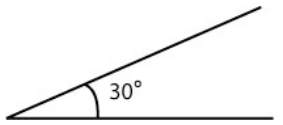This angle measures 30º This angle measure is less than 90º Angles less than 90º are called acute angles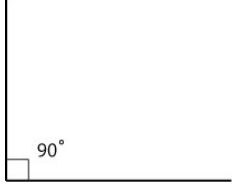This angle measures 90º Angles that measure exactly 90º are called right angles The small box in the angle corner denotes a right angle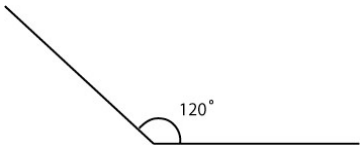This angle measures 120º This angle measure is more than 90º Angles that measure more than 90º but less 180º than are called obtuse angles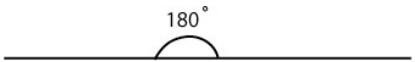This angle measures 180º Angles that measure exactly 180º are called straight angles